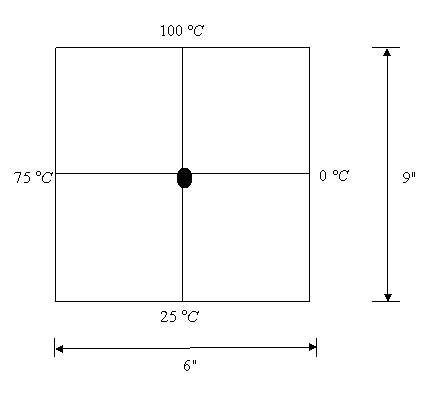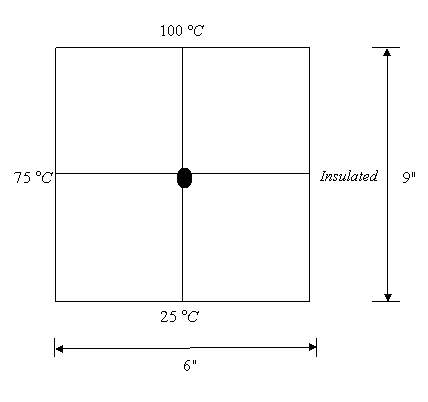# Quiz Chapter 10.03: Elliptic Partial Differential Equations

 MULTIPLE CHOICE TEST ELLIPTIC PARTIAL DIFFERENTIAL EQUATIONS PARTIAL DIFFERENTIAL EQUATIONS

1. In a general second order linear partial differential equation with two independent variables,

• $A \dfrac{\partial^{2} u}{\partial x^{2}} + B \dfrac{\partial^{2} u}{\partial x \partial y} + C \dfrac{\partial^{2} u}{\partial y^{2}} + D = 0$

Where $A, \, B, \, C$ are functions of $x$ and $y$, and $D$ is a function of $x, \, y, \, \dfrac{\partial u}{\partial x}, \, \dfrac{\partial u}{\partial y}$, then the PDE is elliptic if

2. The region in which the following equation

• $x^{3} \dfrac{\partial^{2} u}{\partial x^{2}} + 27 \dfrac{\partial^{2} u}{\partial y^{2}} + 3 \dfrac{\partial^{2} u}{\partial x \partial y} + 5u = 0$

acts as a parabolic equation is

3. The finite difference approximation of $\dfrac{\partial^{2} u}{\partial x^{2}}$ in the elliptic equation

• $\dfrac{\partial^{2} u}{\partial x^{2}} + \dfrac{\partial^{2} u}{\partial y^{2}} = 0$

at $(x, \, y)$ can be approximated as

4. Find the temperature at the interior node given in the following figure using the direct method5. Find the temperature at the interior node given in the following figureUsing the Lieberman method and relaxation factor of $1.2$, the temperature at $x=3, \, y=6$ estimated after $2$ iterations is (use the temperature of interior nodes as $50^{\circ}$C for the initial guess)

6. Find the steady-state temperature at the interior node as given in the following figure